## Name

ST_Buffer — 计算覆盖距几何体给定距离内所有点的几何体。

## Synopsis

`geometry ST_Buffer(`geometry g1, float radius_of_buffer, text buffer_style_parameters = ''`)`;

`geometry ST_Buffer(`geometry g1, float radius_of_buffer, integer num_seg_quarter_circle`)`;

`geography ST_Buffer(`geography g1, float radius_of_buffer, text buffer_style_parameters`)`;

`geography ST_Buffer(`geography g1, float radius_of_buffer, integer num_seg_quarter_circle`)`;

## 描述

• 'endcap=round|flat|square' ：endcap 样式（默认为“round”）。 “butt”被认为是“flat”的同义词。

• 'join=round|mitre|bevel' ：连接样式（默认为“round”）。 “mitre”被认为是“mitre”的同义词。

• 'mitre_limit=#.#' ：斜接比率限制（仅影响斜接连接样式）。 “miter_limit”被接受为“mitre_limit”的同义词。

• 'side=both|left|right' ：'left' 或 'right' 在几何体上执行单边缓冲，缓冲边相对于线的方向。 这仅适用于 LINESTRING 几何图形，不影响 POINT 或 POLYGON 几何图形。 默认情况下，终止样式是方形的。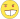对于地理数据，这是对几何数据实现的一个轻量级封装。 它确定最适合地理对象边界框的平面空间参考系统（尝试 UTM、兰伯特方位角等积 (LAEA) 北/南极，最后是墨卡托）。 缓冲区在平面空间中计算，然后转换回 WGS84。 如果输入对象远大于 UTM 区域或跨越日期变更线，这可能不会产生所需的行为缓冲区输出始终是有效的多边形几何体。 缓冲区可以处理无效输入，因此距离 0 的缓冲有时被用作修复无效多边形的一种方法。 ST_MakeValid也可用于此目的。缓冲有时用于执行近距离搜索。 对于此用例，使用ST_DWithin效率更高。此函数忽略 Z 维度。 即使在 3D 几何体上使用时，它也始终给出 2D 结果。此方法实现了 SQL 1.1 的 OGC 简单功能规范。 s2.1.1.3该方法实现了SQL/MM规范。 SQL-MM IEC 13249-3: 5.1.30

## 示例quad_segs=8 (默认) ```SELECT ST_Buffer( ST_GeomFromText('POINT(100 90)'), 50, 'quad_segs=8'); ```quad_secs=2（不足） ```SELECT ST_Buffer( ST_GeomFromText('POINT(100 90)'), 50, 'quad_segs=2'); ```endcap=round join=round (默认) ```SELECT ST_Buffer( ST_GeomFromText( 'LINESTRING(50 50,150 150,150 50)' ), 10, 'endcap=round join=round'); ```endcap=square ```SELECT ST_Buffer( ST_GeomFromText( 'LINESTRING(50 50,150 150,150 50)' ), 10, 'endcap=square join=round'); ```endcap=flat ```SELECT ST_Buffer( ST_GeomFromText( 'LINESTRING(50 50,150 150,150 50)' ), 10, 'endcap=flat join=round'); ```join=bevel ```SELECT ST_Buffer( ST_GeomFromText( 'LINESTRING(50 50,150 150,150 50)' ), 10, 'join=bevel'); ```join=mitre mitre_limit=5.0 (默认最大斜接比率) ```SELECT ST_Buffer( ST_GeomFromText( 'LINESTRING(50 50,150 150,150 50)' ), 10, 'join=mitre mitre_limit=5.0'); ```join=mitre mitre_limit=1 ```SELECT ST_Buffer( ST_GeomFromText( 'LINESTRING(50 50,150 150,150 50)' ), 10, 'join=mitre mitre_limit=1.0'); ```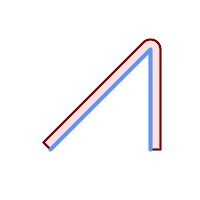side=left ```SELECT ST_Buffer( ST_GeomFromText( 'LINESTRING(50 50,150 150,150 50)' ), 10, 'side=left'); ```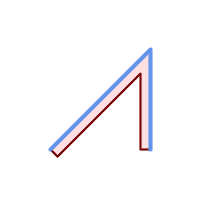side=right ```SELECT ST_Buffer( ST_GeomFromText( 'LINESTRING(50 50,150 150,150 50)' ), 10, 'side=right'); ```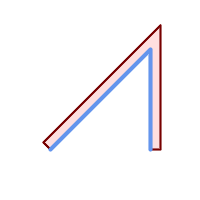side=left join=mitre ```SELECT ST_Buffer( ST_GeomFromText( 'LINESTRING(50 50,150 150,150 50)' ), 10, 'side=left join=mitre'); ```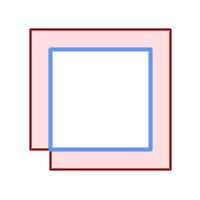顺时针方向，多边形边界向左 ```SELECT ST_Buffer( ST_ForceRHR( ST_Boundary( ST_GeomFromText( 'POLYGON ((50 50, 50 150, 150 150, 150 50, 50 50))'))), ), 20, 'side=left'); ```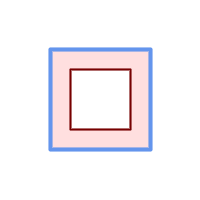顺时针方向，多边形边界向右 ```SELECT ST_Buffer( ST_ForceRHR( ST_Boundary( ST_GeomFromText( 'POLYGON ((50 50, 50 150, 150 150, 150 50, 50 50))')) ), 20,'side=right') ```
```--A buffered point approximates a circle
-- A buffered point forcing approximation of (see diagram)
-- 2 points per quarter circle is poly with 8 sides (see diagram)
SELECT ST_NPoints(ST_Buffer(ST_GeomFromText('POINT(100 90)'), 50)) As promisingcircle_pcount,
ST_NPoints(ST_Buffer(ST_GeomFromText('POINT(100 90)'), 50, 2)) As lamecircle_pcount;

promisingcircle_pcount | lamecircle_pcount
------------------------+-------------------
33 |                9

--A lighter but lamer circle
-- only 2 points per quarter circle is an octagon
--Below is a 100 meter octagon
-- Note coordinates are in NAD 83 long lat which we transform
to Mass state plane meter and then buffer to get measurements in meters;
SELECT ST_AsText(ST_Buffer(
ST_Transform(
ST_SetSRID(ST_Point(-71.063526, 42.35785),4269), 26986)
,100,2)) As octagon;
----------------------
POLYGON((236057.59057465 900908.759918696,236028.301252769 900838.049240578,235
957.59057465 900808.759918696,235886.879896532 900838.049240578,235857.59057465
900908.759918696,235886.879896532 900979.470596815,235957.59057465 901008.759918
696,236028.301252769 900979.470596815,236057.59057465 900908.759918696))
```